# CelesteCS Math Plugin: Working with Tables

Working with tables by referencing their values from the formulas is the most often used functionality of CelesteCS Math plugin. This part of work is similar for Math Server and Math Cloud plugins.

The following article will show the common usage scenario of table processing in Math plugin.

1.Start creating a new page or editing an existing one.

•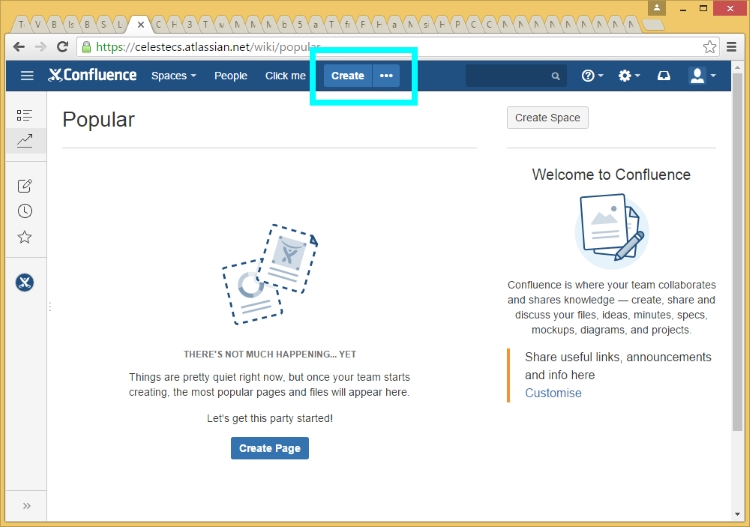2.We will start from an empty page to avoid any not needed details.

•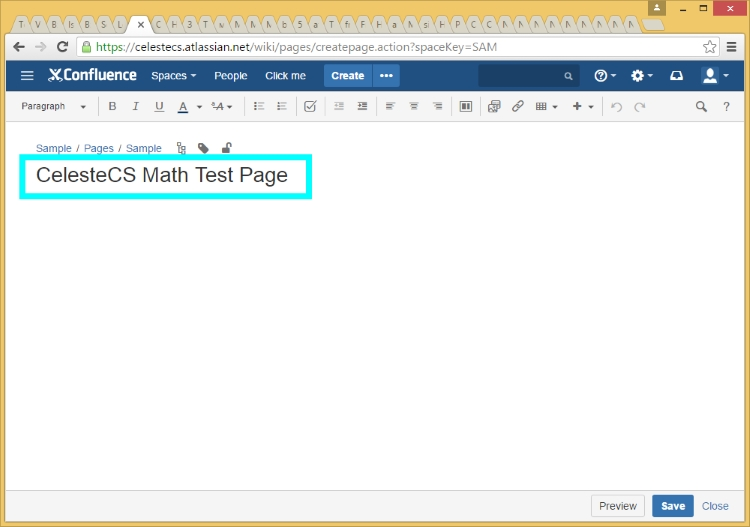3.Add a couple of tables with numerical values. Note, that Math plugin supports numerical, logical, date and text values. If cell contains some text with a number, this number may be retrieved with VALUE function, for example VALUE(‘Line 123’) will return a number 123.

•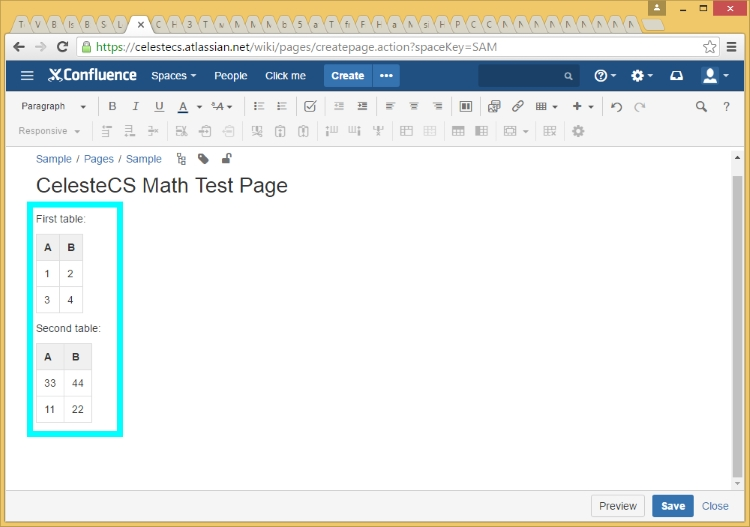4.Now let’s add Math macro to the page. Click “+” button and choose “Numerical Expression Cloud” macro. If it is not shown in the menu, click “Other macros” and find it in the full list of macros. Numerical Expression Macro is the main part of Math plugin, which provides formula calculation functionality.

•5.Configure macro to read outer table values from the current page by setting the ‘Outer values source’ parameter to ‘Page content’. Other supported variants are ‘Macro body’, when values are searched for in the macro body and ‘Other page’, when the values are taken from the page, specified in ‘Other Confluence page’ parameter. Only one source is allowed at a time.

•6.After configuring a macro close the dialog and insert the following simple formula into the macro placeholder window: ‘Table1.A2 + Table2.B3’. Let’s go through the formula parts. Tables on a page (or macro body) are indexed starting from 1, so we are referencing the first table (‘Table1’) and the second one (Table2). Referencing table cell is similar to Excel, where letters mean column index and number means row index, starting from 1. As both referenced values are numerical values, their sum will be a sum of two numbers.

•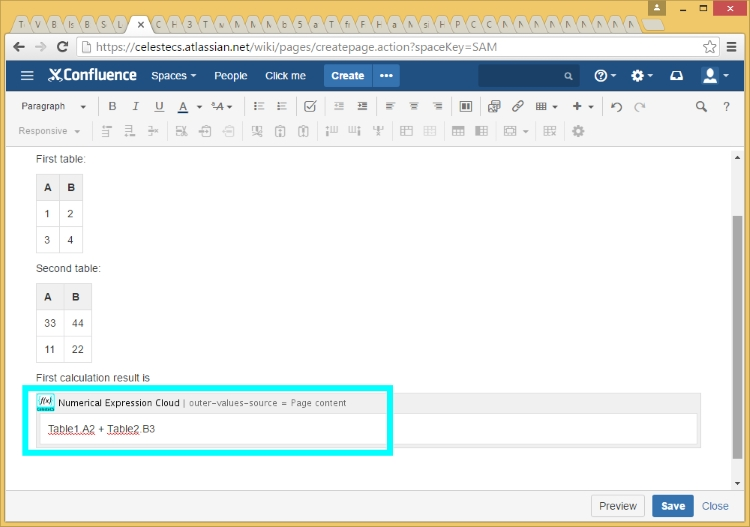7.Save the page and here is the result of calculation (Table1.A2 + Table2.B3 = 1 + 22 = 23):

•8.That was too simple. Let’s go further. You may specify a paragraph of text in a macro body with enclosing formula in curly or square brackets. That is controlled with ‘Macro body content’ parameter, which may have one of three values: ‘Single expression’ means whole macro body contents will be processed as a formula; ‘Text and expression in curly braces’ means that the body contents will be searched for the formulas, enclosed in curly braces and will be replaced with the calculation result and the rest text will be copied as-is; ‘Text and expression in square braces’ means that the body contents will be searched for the formulas, enclosed in square braces and will be replaced with the calculation result. Select a variant with curly braces for this example:

•9.Add the following text to the macro body: ‘Second calculation result is { SUM(Table1.A2:B3) }’.

•10.After saving the page you will see the following result:

•11.And here is the last example in this article. Copy and paste previous macro and replace old text in the macro body with the following one: ‘Third calculation result is { SUM(Table1.A2:B3) / MIN(Table2.A2:B3) }’.

•12.Save the page and you will see the following result:

•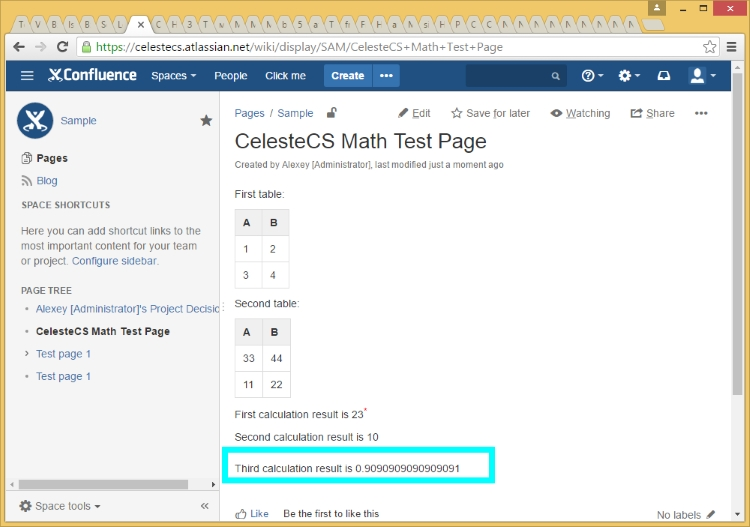13.This ‘9090909090’ looks weird. Let’s apply ROUND function here. Replace the macro body text with the following one: ‘Third calculation result is { ROUND(100 * SUM(Table1.A2:B3) / MIN(Table2.A2:B3)) / 100 }’.

•14.When you save the page, you will see the result, which is much better:

•Notes:

1.Red star right after calculation result means, that an evaluation version of Math macro is used. It disappears when applying a commercial or developer license.

2.There are much more functions, supported by the plugin. Feel free to read Numerical Expression Macro Reference.